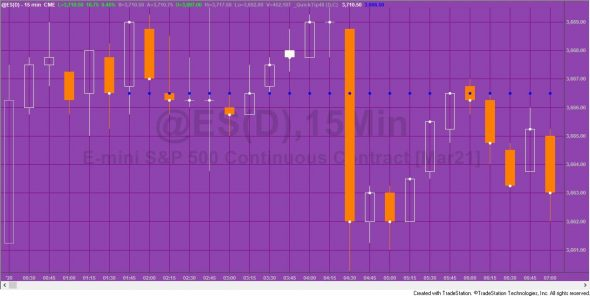# Quick-tip 48 | The behavior of reserved words in inputs and variables

Quick-tip 48 examines the behavior of putting reserved words: Date (D) and Close (C) in inputs and variables as in the following syntax:

```Input: DateInput( D ),
CloseInput( C );

Vars: DateVar( D ),
CloseVar( C );

Print( D, " ", T," DateInput ",DateInput," DateVar ",DateVar );

Plot1( CloseInput,"CloseInp", White );
Plot2( CloseVar, "CloseVar", Blue );```

The results of the plots can be seen in the following image:The blue dots are a plot of the variable. The white dots are a plot of the input.
The same sort of behavior described in this QuickTip would also apply to reserved words: Time, Volume, Low, High etc